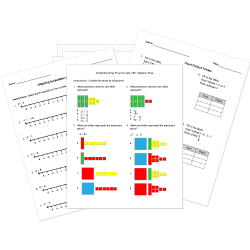Looking for Algebra worksheets?
Check out our pre-made Algebra worksheets!
 Tweet##### Browse Questions
• Arts (209)
• English Language Arts (2742)
• English as a Second Language ESL (1382)
• Health and Medicine (396)
• Life Skills (593)
• Math (2309)

• ### Vectors

• #### Trigonometry

• Physical Education (200)
• Science (3784)
• Social Studies (1794)
• Study Skills and Strategies (15)
• Technology (549)
• Vocational Education (648)

You can create printable tests and worksheets from these Grade 11 Algebraic Expressions questions! Select one or more questions using the checkboxes above each question. Then click the add selected questions to a test button before moving to another page.

Grade 11 Algebraic Expressions CCSS: HSA-SSE.A.2
Which of the expressions below is equivalent to $(3x+5)(2x-2)$ ?
1. $6x^2+10x-10$
2. $3x(2x-2)+5(2x-2)$
3. $2x(3x+5)+2(3x+5)$
4. $3x^2-4x-10$
Grade 11 Algebraic Expressions CCSS: HSA-SSE.A.2
Which of the expressions below is equivalent to $(6x^2+2y)^2*2(3x^2+y)$ ?
1. $36x^4+4y^4+6x^2+2y$
2. $12x^2+4y$
3. $(6x^2+2y)^3$
4. $42x^2+6y^2$
Grade 11 Algebraic Expressions CCSS: HSA-SSE.A.2
Which of the expressions below is equivalent to $4x^2-4y^2$ ?
1. $(2x-2y)(2x+2y)$
2. $(4x-4y)(4x+4y)$
3. $(2x-2y)(2x-2y)$
4. $(x-y)(x-y)$
Grade 11 Algebraic Expressions CCSS: HSA-SSE.A.2
Which of the expressions below is equivalent to $4x^2-12x+9$ ?
1. $(2x+3)(2x-3)$
2. $"No" \ "Answer"$
3. $(2x+3)^2$
4. $2x(2x-3)-3(2x-3)$
Grade 11 Algebraic Expressions CCSS: HSA-SSE.A.2
Which of the expressions below is equivalent to $5(x-2y)-3x(-2y+x)$ ?
1. $(5-3x)(x-2y)$
2. $-15x(x-2y)$
3. $-15x(x-2y)(-2y+x)$
4. $-15x(x-2y)^2$